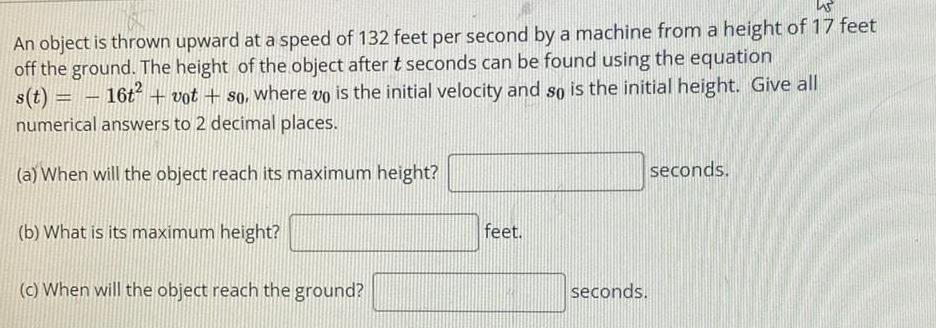Question:

# An object is thrown upward at a speed of 132 feet per second

Last updated: 8/14/2022An object is thrown upward at a speed of 132 feet per second by a machine from a height of 17 feet off the ground. The height of the object after t seconds can be found using the equation s(t) = 16t² + vot + so, where is the initial velocity and so is the initial height. Give all numerical answers to 2 decimal places. (a) When will the object reach its maximum height? (b) What is its maximum height? (c) When will the object reach the ground?